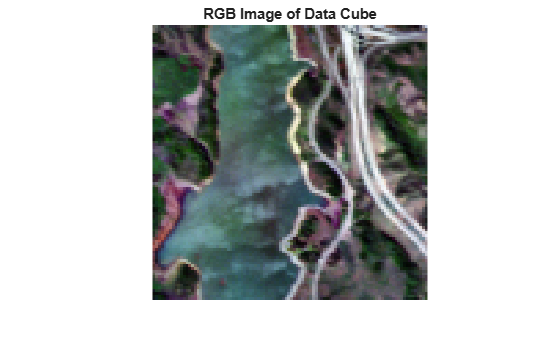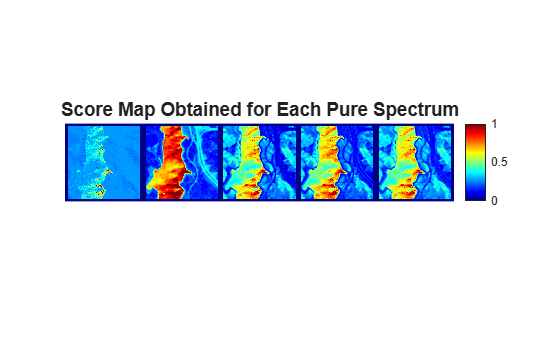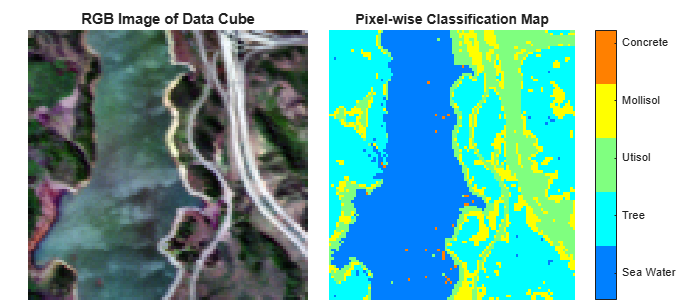# Classify Hyperspectral Image Using Library Signatures and SAM

This example shows how to classify pixels in a hyperspectral image by using the spectral angle mapper (SAM) classification algorithm. This algorithm classifies each pixel in the test data by computing the spectral match score between the spectrum of a pixel and the pure spectral signatures read from the ECOSTRESS spectral library. This example uses a data sample from the Jasper Ridge dataset as the test data. The test data contains four endmembers latent, consisting of roads, soil, water, and trees. In this example, you will:

1. Generate a score map for different regions present in the test data by computing the SAM spectral match score between the spectrum of each test pixel and a pure spectrum. The pure spectra are from the ECOSTRESS spectral library.

2. Classify the regions by using minimum score criteria, and assign a class label for each pixel in the test data.

Read test data from the Jasper Ridge dataset by using the `hypercube` function. The function returns a `hypercube` object, which stores the hyperspectral data cube and the corrsponding wavelength and metadata information read from the test data. The test data has 198 spectral bands and their wavelengths range from 399.4 nm to 2457 nm. The spectral resolution is up to 9.9 nm and the spatial resolution of each band image is 100-by-100.

`hcube = hypercube('jasperRidge2_R198.img')`
```hcube = hypercube with properties: DataCube: [100×100×198 int16] Wavelength: [198×1 double] Metadata: [1×1 struct] ```

Estimate an RGB image from the data cube. Apply contrast stretching to enhance the contrast of the output RGB image.

`rgbImg = colorize(hcube,'Method','rgb','ContrastStretching',true);`

Display the RGB image of the test data.

```figure imagesc(rgbImg); axis image off title('RGB Image of Data Cube')```### Read Signatures from ECOSTRESS Spectral Library

The ECOSTRESS spectral ibrary consists of pure spectral signatures for individual surface materials. If the spectrum of a pixel matches a signature from the ECOSTRESS library, the pixel consists entirely of that single surface material. The library is a compilation of over 3400 spectral signatures for both natural and manmade materials. Since you know the the endmembers latent in the test data, choose the ECOSTRESS spectral library files related to those four endmembers.

Read spectral files related to water, vegetation, soil, and concrete from the ECOSTRESS spectral library. Use the spectral signatures of these types:

• Soil to classify sand, silt, and clay regions

• Vegetation to classify tree regions

• Water to classify water regions

```fileroot = matlabshared.supportpkg.getSupportPackageRoot(); addpath(fullfile(fileroot,'toolbox','images','supportpackages','hyperspectral',... 'hyperdata','ECOSTRESSSpectraFiles')); filenames = ["water.seawater.none.liquid.tir.seafoam.jhu.becknic.spectrum.txt",... "vegetation.tree.eucalyptus.maculata.vswir.jpl087.jpl.asd.spectrum.txt",... "soil.utisol.hapludult.none.all.87p707.jhu.becknic.spectrum.txt",... "soil.mollisol.cryoboroll.none.all.85p4663.jhu.becknic.spectrum.txt",... "manmade.concrete.pavingconcrete.solid.all.0092uuu_cnc.jhu.becknic.spectrum.txt"]; lib = readEcostressSig(filenames)```
```lib=1×5 struct array with fields: Name Type Class SubClass ParticleSize Genus Species SampleNo Owner WavelengthRange Origin CollectionDate Description Measurement FirstColumn SecondColumn WavelengthUnit DataUnit FirstXValue LastXValue NumberOfXValues AdditionalInformation Wavelength Reflectance ⋮ ```

Extract the class names from the library structure.

`classNames = [lib.Class];`

Plot the pure spectral signatures read from the ECOSTRESS spectral library.

```figure hold on for idx = 1:numel(lib) plot(lib(idx).Wavelength,lib(idx).Reflectance,'LineWidth',2) end axis tight box on title('Pure Spectral Signatures from ECOSTRESS Library') xlabel('Wavelength (\mum)') ylabel('Reflectance (%)') legend(classNames,'Location','northeast') title(legend,'Class Names') hold off```### Compute Score Map for Pixels in Test Data

Find the spectral match score between each pixel spectrum and the library signatures by using the `spectralMatch` function. By default, the `spectralMatch` function computes the degree of similarity between two spectra by using the SAM classification algorithm. The function returns an array with the same spatial dimensions as the hyperspectral data cube and channels equal to the number of library signatures specified. Each channel contains the score map for a single library signature. In this example, there are five ECOSTRESS spectral library files specified for comparison, and each band of the hyperspectral data cube has spatial dimensions of 100-by-100 pixels. The size of the output array of score maps thus is 100-by-100-by-5.

`scoreMap = spectralMatch(lib,hcube);`

Display the score maps.

```figure montage(scoreMap,'Size',[1 numel(lib)],'BorderSize',10) title('Score Map Obtained for Each Pure Spectrum','FontSize',14) colormap(jet); colorbar```### Classify Pixels Using Minimum Score Criteria

Lower SAM values indicate higher spectral similarity. Use the minimum score citeria to classify the test pixels by finding the best match for each pixel among the library signatures. The result is a pixel-wise classification map in which the value of each pixel is the index of library signature file in `lib` for which that pixel exhibits the lowest SAM value. For example, if the value of a pixel in the classification map is 1, the pixel exhibits high similarity to the first library signature in `lib`.

`[~,classMap] = min(scoreMap,[],3);`

Create a class table that maps the classification map values to the ECOSTRESS library signatures used for spectral matching.

```classTable = table((min(classMap(:)):max(classMap(:)))',classNames',... 'VariableNames',{'Classification map value','Matching library signature'})```
```classTable=5×2 table Classification map value Matching library signature ________________________ __________________________ 1 "Sea Water" 2 "Tree" 3 "Utisol" 4 "Mollisol" 5 "Concrete" ```

Display the RGB image of the hyperspectral data and the classification results. Visual inspection shows that spectral matching classifies each pixel effectively.

```fig = figure('Position',[0 0 700 300]); axes1 = axes('Parent',fig,'Position',[0.04 0 0.4 0.9]); imagesc(rgbImg,'Parent',axes1); axis off title('RGB Image of Data Cube') axes2 = axes('Parent',fig,'Position',[0.47 0 0.45 0.9]); imagesc(classMap,'Parent',axes2) axis off colormap(jet(numel(lib))) title('Pixel-wise Classification Map') ticks = linspace(1.4,4.8,numel(lib)); colorbar('Ticks',ticks,'TickLabels',classNames) ```#### References

 Kruse, F.A., A.B. Lefkoff, J.W. Boardman, K.B. Heidebrecht, A.T. Shapiro, P.J. Barloon, and A.F.H. Goetz. “The Spectral Image Processing System (SIPS)—Interactive Visualization and Analysis of Imaging Spectrometer Data.” Remote Sensing of Environment 44, no. 2–3 (May 1993): 145–63. https://doi.org/10.1016/0034-4257(93)90013-N.